# Operators in Python

## Operators in Python with Examples

In this article, I am going to discuss Operators in Python with Examples. Please read our previous article where we discussed Data Types in Python with examples. At the end of this article, you will understand the following pointers in detail.

1. What is an operator?
2. Different type of operators
3. Arithmetic Operators
4. Assignment operator
5. Unary minus operator
6. Relational operators
7. Logical operators
8. Membership operators
9. Identity operators
10. Multiple Programs to understand the above concepts
##### What is an Operator?

In programming languages, an operator is a symbol that is applied to some operands (usually variables), to perform certain actions or operations. For example,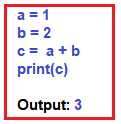In the above example, first, we assigned values to two variables ‘a’ and ‘b’ and then we added both the variables and stored the result in variable ‘c’. The operations we performed are:

1. Assignment Operation using ‘=’ operator. We assigned the values to variables ‘a’, ‘b’, ‘c’ using the operator ‘=’ which is called the assignment operator.
2. Addition Operation using ‘+’ operator. The values in variables are added using the ‘+’ operator.

Note: The symbols + and = are called operators and the variables a,b,c on which operation is being performed are called operands.

##### BASIC CLASSIFICATION OF OPERATORS IN PYTHON

Unary Operator – If the operator acts on a single operand then it’s called the unary operator. For example, in ‘-5’ the operator ‘-’ is used to make the (single) operand ‘5’ a negative value hence called the unary operator.

Binary Operator – If the operator acts on two operands then it’s called a binary operator. For example, + operators need two variables(operands) to perform some operation, hence called binary operator.

Ternary Operator – If the operator acts on three operands then it’s called a ternary operator. In general, the ternary operator is a precise way of writing conditional statements. The concept behind this will be understood in the later chapters. For now, just consider the simple syntax shown below.

x = true_value if condition else false_value

The three operands here are :

1. condition
2. true_value
3. False_value

Here if the condition is evaluated to true then the value in true_value will be in x else the value in false_value.

##### Types Of Operators in Python:
1. Arithmetic Operators: The operators which are used to perform arithmetic operations like addition, subtraction, division etc. They are: +, -, *, /, %, **, //
2. Relational Operators: The operators which are used to check for some relation like greater than or less than etc.. between operands are called relational operators. They are: <, >, <=, >=, ==, !=
3. Logical Operators: The operators which do some logical operation on the operands and return True or False are called logical operators. The logical operations can be ‘AND’, ‘OR’ etc.
4. Assignment Operators: The operators which are used for assigning values to variables. ‘=’ is the assignment operator.
5. Unary Minus Operator: The operator ‘-’ is called the Unary minus operator. It is used to negate the number.
6. Membership Operators: The operators that are used to check whether a particular variable is part of another variable or not. They are ‘in’ and ‘not in’
7. Identity Operators: The operators which are used to check for identity. They are ‘is’ and ‘is not’.
##### ARITHMETIC OPERATORS IN PYTHON:

As stated above, these are used to perform that basic mathematical stuff as done in every programming language. Let’s understand them with some examples. Let’s assume, a = 20 and b = 12Note: Division operator / always performs floating-point arithmetic, so it returns a float value. Floor division (//) can perform both floating-point and integral as well,

1. If values are int type, the result is int type.
2. If at least one value is float type, then the result is of float type.
###### Example: Arithmetic Operators in Python
```a = 20
b = 12
print(a+b)
print(a-b)
print(a*b)
print(a/b)
print(a%b)
print(a**b)
print(a//b)```
###### Output: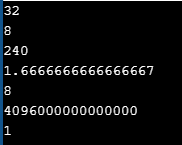###### Example: Floor division
```print(12//5)
print(12.0//5)```

Output:
2
2.0

##### RELATIONAL OPERATORS IN PYTHON:

These are used to compare two values for some relation and return True or False depending on the relation. Let’s assume, a = 13 and b = 5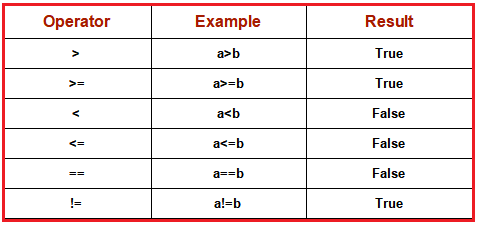Relational operators are used, in general, for construction simple conditions.

##### Example: Relational Operators in Python
```a = 13
b = 5
print(a>b)
print(a>=b)
print(a<b)
print(a<=b)
print(a==b)
print(a!=b)```
###### Output: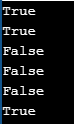##### LOGICAL OPERATORS IN PYTHON

In python, there are three types of logical operators. They are and, or, not. These operators are used to construct compound conditions, combinations of more than one simple condition. Each simple condition gives a boolean value which is evaluated, to return the final boolean value.

Note: In logical operators, False indicates 0(zero) and True indicates non-zero value. Logical operators on boolean types

1. and: If both the arguments are True then only the result is True
2. or: If at least one argument is True then the result is True
3. not: the complement of the boolean value
###### Example: Logical operators on boolean types in Python
```a = True
b = False
print(a and b)
print(a or b)
print(not a)
print(a and a)```
###### Output: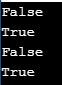##### Logical operators on non-boolean types

Please understand the below statements carefully,

##### and operator:

‘A and B’ returns A if A is False

‘A and B’ returns B if A is not False

###### Examples: ( 0 means False and non-zero means True)
1. 0 and 4 returns 0
2. 5 and 7 returns 7
3. 21 and 0 returns 0
4. 15 and 8 returns 8
##### Example: Logical and operators on non-boolean types in Python
```print(0 and 4)
print(5 and 7)
print(21 and 0)
print(15 and 8)```

Output:
0
7
0
8

###### Example: Logical and operators on non-boolean types in python
```x = 0
y = 4
a = 4
b = 7

print(x and y)
print(a and b)```

Output:
0
7

###### Example: Logical and operators on non-boolean types in python
```x = 21
y = 0
a = 15
b = 8
print(x and y)
print(a and b)```

Output:
0
8

##### Or Operator in Python:

‘A or B’ returns A if A is True

‘A or B’ returns B if A is not True

###### Examples: (0 means False and non-zero means True)
1. 0 or 4 returns 4
2. 5 or 7 returns 7
3. 21 or 0 returns 21
4. 15 or 8 returns 8
###### Example: Logical OR operators on non-boolean types in python
```print(0 or 4)
print(5 or 7)
print(21 or 0)
print(15 or 8)```
###### Output: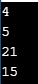```x = 0
y = 4
a = 5
b = 7

print(x or y)
print(a or b)```

Output: 4 5

###### Example: Logical or operators on non-boolean types in python
```x = 21
y = 0
a = 15
b = 8

print(x or y)
print(a or b)```

Output: 21 15

##### Not Operator in Python:

not A returns False if A is True

not B returns True if A is False

###### Examples, (0 means False and non-zero means True)
1. not 5 returns False
2. not 0 returns True
###### Example: Logical not operator on non-boolean types in python
```print(not 5)
print(not 0)```

Output:
False
True

```x = 5
y = 0
print(not x)
print(not y)```

Output:
False
True

##### Conclusion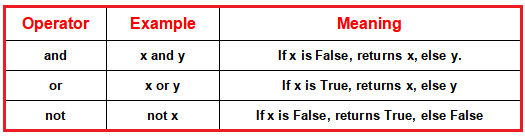##### ASSIGNMENT OPERATORS IN PYTHON

By using these operators, we can assign values to variables. ‘=’ is the assignment operator used in python. There are some compound operators which are the combination of some arithmetic and assignment operators (+=, -=, *=, /=, %=, **=, //= ). Assume that, a = 13 and b = 5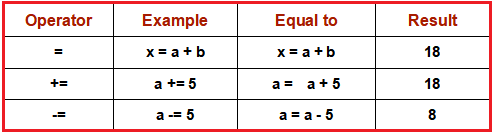```a=13
print(a)
a+=5
print(a)```

Output:
13
18

##### UNARY MINUS OPERATOR(-) IN PYTHON:

This operator operates on a single operand, hence unary operator. This is used to change a positive number to a negative number and vice-versa.

```a=10
print(a)
print(-a)```

Output:
10
-10

##### MEMBERSHIP OPERATORS IN PYTHON

Membership operators are used to checking whether an element is present in a sequence of elements are not. Here, the sequence means strings, list, tuple, dictionaries, etc which will be discussed in later chapters. There are two membership operators available in python i.e. in and not in.

1. in operator: The in operators returns True if element is found in the collection of sequences. returns False if not found
2. not in operator: The not-in operator returns True if the element is not found in the collection of sequence. returns False in found
###### Example: Membership Operators in Python
```text = "Welcome to python programming"
print("Welcome" in text)
print("welcome" in text)
print("nireekshan" in text)
print("Hari" not in text)```
###### Output: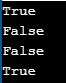###### Example: Membership Operators in Python
```names = ["Ramesh", "Nireekshan", "Arjun", "Prasad"]
print("Nireekshan" in names)
print("Hari" in names)
print("Hema" not in names)```
###### Output: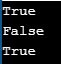##### IDENTITY OPERATOR IN PYTHON

This operator compares the memory location( address) to two elements or variables or objects. With these operators, we will be able to know whether the two objects are pointing to the same location or not. The memory location of the object can be seen using the id() function.

###### Example: Identity Operators in Python
```a = 25
b = 25
print(id(a))
print(id(b))```
###### Output: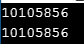##### Types of Identity Operators in Python:

There are two identity operators in python, is and is not.

###### is:
1. A is B returns True, if both A and B are pointing to the same address.
2. A is B returns False, if both A and B are not pointing to the same address.
###### is not:
1. A is not B returns True, if both A and B are not pointing to the same object.
2. A is not B returns False, if both A and B are pointing to the same object.
###### Example: Identity Operators in Python
```a = 25
b = 25
print(a is b)
print(id(a))
print(id(b))```
###### Output: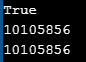###### Example: Identity Operators
```a = 25
b = 30
print(a is b)
print(id(a))
print(id(b))```
###### Output: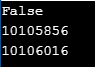Note: The ‘is’ and ‘is not’ operators are not comparing the values of the objects. They compare the memory locations (address) of the objects. If we want to compare the value of the objects, we should use the relational operator ‘==’.

###### Example: Identity Operators
```a = 25
b = 25
print(a == b)```

Output: True

###### Example: Identity Operators
```a = 25
b = 30
print(a == b)```

Output: False